江淮 同悦RS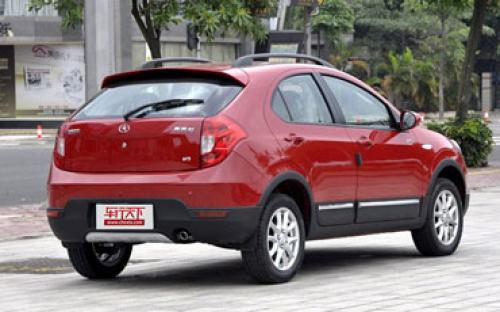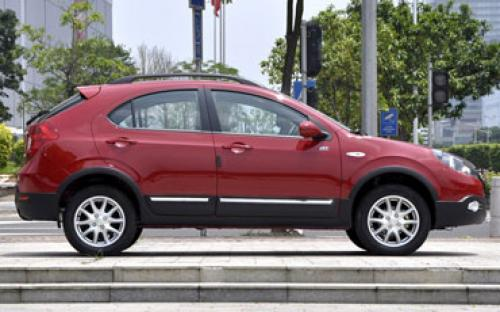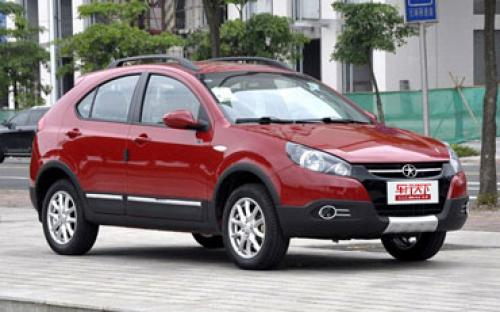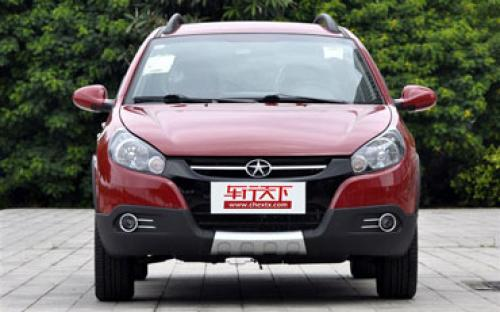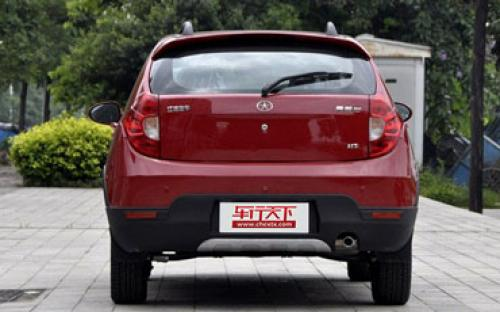9 种颜色可选2012款最低售价：4.88 万元起

3965(mm)1650(mm)1445(mm)配置亮点：
• 胎压监测装置

• ISOFIX儿童座椅接口

• 车身稳定控制(ESC/ESP/DSC等)

• 电动天窗

• 定速巡航

• 后倒车雷达

• 真皮座椅

• GPS导航系统

• 氙气大灯

• 后视镜加热江淮 同悦RS 在售车型

排量 车型 厂商指导价 本地最低报价 购车工具
1.3L RS 1.3L 手动标准型 停产停售 5挡手动 4.88万 4.88万

询底价+对比
RS 1.3L 手动舒适型 停产停售 5挡手动 5.08万 5.08万

询底价+对比
RS 1.3L 手动豪华型 停产停售 5挡手动 5.38万 5.38万

询底价+对比
RS 1.3L 手动尚动豪华型 停产停售 5挡手动 5.48万 5.48万

询底价+对比
RS 1.3L 手动尚动尊贵型 停产停售 5挡手动 5.68万 5.68万

询底价+对比
RS 1.3L AMT豪华型 停产停售 5挡AMT 5.68万 5.68万

询底价+对比
Cross 1.3L 手动舒适型 停产停售 5挡手动 5.38万 5.38万

询底价+对比
Cross 1.3L 手动豪华型 停产停售 5挡手动 5.78万 5.78万

询底价+对比
Cross 1.3L 手动尚动豪华型 停产停售 5挡手动 5.78万 5.78万

询底价+对比
Cross 1.3L 手动尚动尊贵型 停产停售 5挡手动 6.08万 6.08万

询底价+对比
Cross 1.3L AMT豪华型 停产停售 5挡AMT 6.08万 6.08万

询底价+对比

江淮 同悦RS 经销商

查看更多 >>
更多 >>

本地降价排名

江淮 同悦RS 动力加速

同悦RS 0-100公里加速时间分布在 0.0-秒 属于 超跑级

动力级别 加速时间 车型

江淮 同悦RS 视频

江淮 同悦RS 新闻资讯

海滨小试1.3L同悦RS 体验两厢车！

车系文章 超过2888次关注

伴随着青岛难得的晴天，我们对于刚刚进入市场的江淮同悦RS两厢版轿车进行了简单的试驾，这期间编者针对于两厢版进行了后排的全面体验，在抛去了厚重的尾箱后，新车...

测试江淮同悦RS 1.3 MT 家用代步好选择

车系文章 超过3819次关注

之前我们已经对同悦三厢版进行过一次简单的测试，后来这款小车就经常出现在我们的视野中，这说明它在一定程度上得到了消费者的认可。很快厂家又推出了两厢车型，并...

实拍同悦Cross图解 不能只做花瓶

车系文章 超过3017次关注

作为一家以MPV车型为毛利主要来源的汽车厂商，随着市场竞争的白热化，江淮汽车不断对产品结构进行优化。在三厢版和两厢版同悦相继取得成功之后，江淮把产品线拓展至...

江淮同悦RS Cross或于广州车展上市

车系文章 超过2365次关注

江淮汽车在9月的成都车展上带来了多款新车，日前，我们从官方得到消息，车展亮相的同悦RSCross车型即将上市，具体时间编辑猜测为广州车展期间。

售4.88-6.08万 2012款同悦全系正式上市

车系文章 超过2198次关注

近日，江淮2012款同悦全系车型正式上市，市场售价区间为4.88-6.08万元。本次的集体上市，新车主要是对车型进行精简及整体售价下调，并且新增了CROSS车型供消费者选。

江淮同悦新款VVT发动机即将换装 图片曝光

车系文章 超过2325次关注

此前早有消息，江淮有可能为同悦车型引入一款新的自主发动机，而今日我们捕获了这款新发动机的谍照，据消息人士透露，新款发动机即将替换目前的同悦1.3L机型，最快...

猜你喜欢

﻿
• 快速找车
• 选择品牌
• 选择品牌
• A  奥迪
• A  阿斯顿·马丁
• A  阿尔法·罗密欧
• B  宝沃
• B  布加迪
• B  巴博斯
• B  保时捷
• B  宾利
• B  奔驰
• B  宝马
• B  本田
• B  别克
• B  标致
• B  比亚迪
• B  宝骏
• B  北汽制造
• B  北汽新能源
• B  北汽幻速
• B  北汽威旺
• B  北京汽车
• B  奔腾
• B  北汽绅宝
• C  长安
• C  长安商用
• C  长城
• C  昌河
• D  大众
• D  道奇
• D  DS
• D  东南
• D  东风风神
• D  东风风行
• D  东风小康
• D  东风风度
• D  东风
• F  福特
• F  丰田
• F  菲亚特
• F  法拉利
• F  福田
• F  福迪
• F  福汽启腾
• G  观致
• G  广汽传祺
• G  广汽吉奥
• G  GMC
• H  红旗
• H  汉腾汽车
• H  哈弗
• H  哈飞
• H  海格
• H  海马
• H  华颂
• H  黄海
• H  华泰
• H  恒天
• J  吉利汽车
• J  捷豹
• J  Jeep
• J  江淮
• J  江铃
• J  金杯
• J  九龙
• J  金旅
• K  凯翼
• K  凯迪拉克
• K  克莱斯勒
• K  科尼塞克
• K  卡威
• K  开瑞
• L  路虎
• L  林肯
• L  劳斯莱斯
• L  兰博基尼
• L  雷克萨斯
• L  铃木
• L  雷诺
• L  理念
• L  力帆
• L  莲花汽车
• L  猎豹
• L  路特斯
• L  陆风
• M  马自达
• M  MG
• M  MINI
• M  玛莎拉蒂
• M  摩根
• M  迈凯轮
• N  纳智捷
• O  欧宝
• O  讴歌
• O  欧朗
• Q  奇瑞
• Q  起亚
• Q  启辰
• R  日产
• R  荣威
• R  瑞麒
• S  三菱
• S  斯威汽车
• S  萨博
• S  smart
• S  斯柯达
• S  斯巴鲁
• S  思铭
• S  双龙
• S  上汽大通
• S  双环
• T  特斯拉
• T  腾势
• W  沃尔沃
• W  五菱汽车
• W  五十铃
• W  威兹曼
• W  威麟
• X  现代
• X  雪佛兰
• X  雪铁龙
• X  西雅特
• Y  一汽
• Y  英菲尼迪
• Y  英致
• Y  依维柯
• Y  野马汽车
• Y  永源
• Z  众泰
• Z  中华
• Z  中兴
• Z  知豆
• 选择车系
• 选择车系
• 车型对比
• 选择品牌
• 选择品牌
• A  奥迪
• A  阿斯顿·马丁
• A  阿尔法·罗密欧
• B  宝沃
• B  布加迪
• B  巴博斯
• B  保时捷
• B  宾利
• B  奔驰
• B  宝马
• B  本田
• B  别克
• B  标致
• B  比亚迪
• B  宝骏
• B  北汽制造
• B  北汽新能源
• B  北汽幻速
• B  北汽威旺
• B  北京汽车
• B  奔腾
• B  北汽绅宝
• C  长安
• C  长安商用
• C  长城
• C  昌河
• D  大众
• D  道奇
• D  DS
• D  东南
• D  东风风神
• D  东风风行
• D  东风小康
• D  东风风度
• D  东风
• F  福特
• F  丰田
• F  菲亚特
• F  法拉利
• F  福田
• F  福迪
• F  福汽启腾
• G  观致
• G  广汽传祺
• G  广汽吉奥
• G  GMC
• H  红旗
• H  汉腾汽车
• H  哈弗
• H  哈飞
• H  海格
• H  海马
• H  华颂
• H  黄海
• H  华泰
• H  恒天
• J  吉利汽车
• J  捷豹
• J  Jeep
• J  江淮
• J  江铃
• J  金杯
• J  九龙
• J  金旅
• K  凯翼
• K  凯迪拉克
• K  克莱斯勒
• K  科尼塞克
• K  卡威
• K  开瑞
• L  路虎
• L  林肯
• L  劳斯莱斯
• L  兰博基尼
• L  雷克萨斯
• L  铃木
• L  雷诺
• L  理念
• L  力帆
• L  莲花汽车
• L  猎豹
• L  路特斯
• L  陆风
• M  马自达
• M  MG
• M  MINI
• M  玛莎拉蒂
• M  摩根
• M  迈凯轮
• N  纳智捷
• O  欧宝
• O  讴歌
• O  欧朗
• Q  奇瑞
• Q  起亚
• Q  启辰
• R  日产
• R  荣威
• R  瑞麒
• S  三菱
• S  斯威汽车
• S  萨博
• S  smart
• S  斯柯达
• S  斯巴鲁
• S  思铭
• S  双龙
• S  上汽大通
• S  双环
• T  特斯拉
• T  腾势
• W  沃尔沃
• W  五菱汽车
• W  五十铃
• W  威兹曼
• W  威麟
• X  现代
• X  雪佛兰
• X  雪铁龙
• X  西雅特
• Y  一汽
• Y  英菲尼迪
• Y  英致
• Y  依维柯
• Y  野马汽车
• Y  永源
• Z  众泰
• Z  中华
• Z  中兴
• Z  知豆
• 选择车系
• 选择车系
• 选择车型
• 选择车型
• 意见反馈